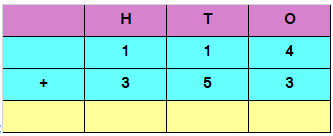a) 467​
b) ​352
c) ​527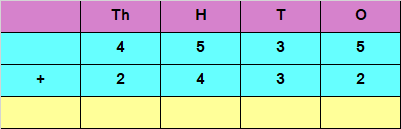a) 7698​
b) ​6967
c) ​5769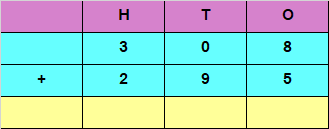a) 730​
b) ​793
c) ​603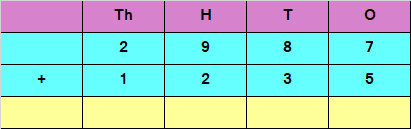a) 4222​
b) ​5632
c) ​5422

Adding the digits​ at ones place,

4 + 3 = 7​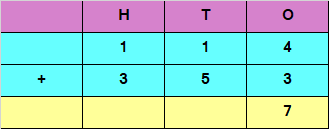​Adding the digits​ at tens place,

1 + 5 = 6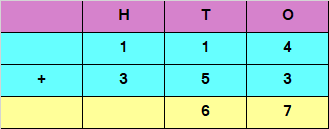​Adding the digits at hundreds place,

1 + 3 = 4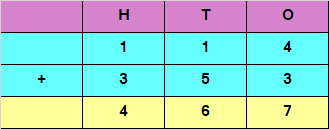Hence, the sum of the given numbers is 467

Adding the digits​ at ones place,

5 + 2 = 7​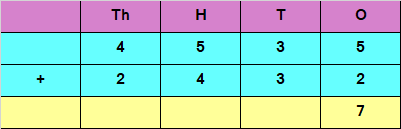​Adding the digits​ at tens place,

3 + 3 = 6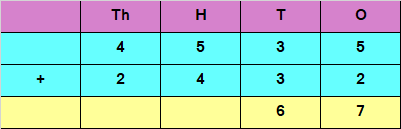​Adding the digits​ at hundreds place,

5 + 4 = 9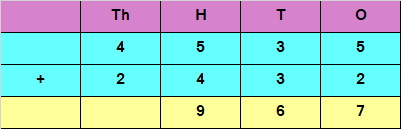​Adding the digits​ at thousands place,

4 + 2 = 6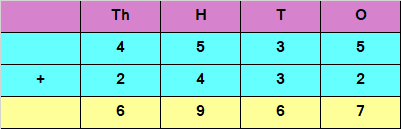​Hence, the sum of the given numbers is 6967

Adding the digits at ones place,

8 + 5 = 13

Since the number is more than 9, we carry over 1​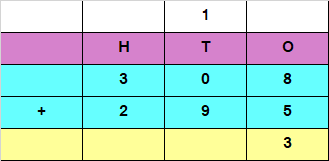​Adding the digits at tens place and the carry of 1

0 + 9 + 1 = 10

Since the number is more than 9, we carry over 1​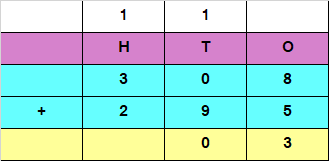​Adding the digits at hundreds place and the carry of 1

3 + 2 + 1 = 6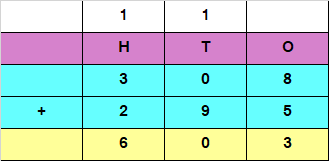Hence, the sum of the given numbers is 603

​Adding the digits at ones place,

7 + 5 = 12

Since the number is more than 9, we carry over 1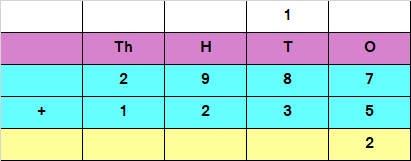​Adding the digits at tens place and the carry of 1

8 + 3 + 1 = 12

Since the number is more than 9, we carry over 1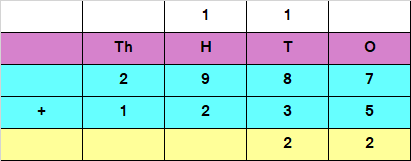​Adding the digits at hundreds place and the carry of 1

9 + 2 + 1 = 12

Since the number is more than 9, we carry over 1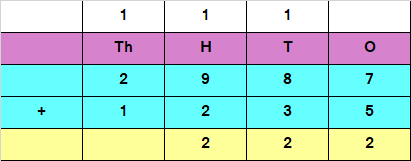​Adding the digits at thousands place and the carry of 1

2 + 1 + 1 = 4

Since the number is more than 9, we carry over 1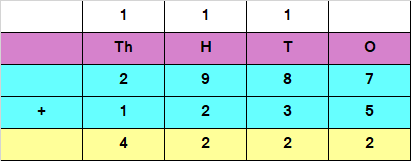​Hence,the sum of the given numbers is 4222